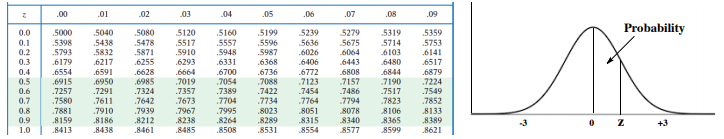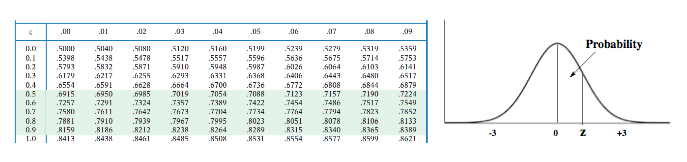Ch.4 + 5 - Statistics, Quality Assurance and Calibration MethodsWorksheetSee all chapters
 Ch.1 - Chemical Measurements 2hrs & 10mins 0% complete Worksheet Ch.2 - Tools of the Trade 1hr & 26mins 0% complete Worksheet Ch.3 - Experimental Error 1hr & 51mins 0% complete Worksheet Ch.4 + 5 - Statistics, Quality Assurance and Calibration Methods 2hrs & 1min 0% complete Worksheet Ch.6 - Chemical Equilibrium 3hrs & 43mins 0% complete Worksheet Ch.7 - Activity and the Systematic Treatment of Equilibrium 1hr & 1min 0% complete Worksheet Ch.8 - Monoprotic Acid-Base Equilibria 1hr & 52mins 0% complete Worksheet Ch.9 - Polyprotic Acid-Base Equilibria 2hrs & 16mins 0% complete Worksheet Ch.10 - Acid-Base Titrations 2hrs & 22mins 0% complete Worksheet Ch.11 - EDTA Titrations 1hr & 37mins 0% complete Worksheet Ch.12 - Advanced Topics in Equilibrium 1hr & 18mins 0% complete Worksheet Ch.13 - Fundamentals of Electrochemistry 2hrs & 27mins 0% complete Worksheet Ch.14 - Electrodes and Potentiometry 55mins 0% complete Worksheet Ch.15 - Redox Titrations 1hr & 14mins 0% complete Worksheet Ch.16 - Electroanalytical Techniques 1hr & 23mins 0% complete Worksheet Ch.17 - Fundamentals of Spectrophotometry 55mins 0% complete Worksheet BONUS: Chemical Kinetics Not available yet

# Hypothesis Testing (t-Test)

See all sections
Sections
Mean Evaluation
The Gaussian Distribution
Confidence Intervals
Hypothesis Testing (t-Test)
Analysis of Variance (f-Test)
Detection of Gross Errors

The t-Test is used to measure the similarities and differences between two populations.

###### The t-test

Concept #1: In order to measure the similarities and differences between populations we utilize a t score

Example #1: A student wishing to calculate the amount of arsenic in cigarettes decides to run two separate methods in her analysis. The results (shown in ppm) are shown below

Sample            Method 1       Method 2

1                      110.5                104.7

2                       93.1                  95.8

3                       63.0                  71.2

4                       72.3                  69.9

5                      121.6                118.7

Is there a significant difference between the two analytical methods under a 95% confidence interval?

Example #2: You want to determine if concentrations of hydrocarbons in seawater measured by fluorescence are significantly different than concentrations measured by a second method, specifically based on the use of gas chromatography/flame ionization detection (GC-FID).  You measure the concentration of a certified standard reference material (100.0 µM) with both methods seven (n=7) times.  Specifically, you first measure each sample by fluorescence, and then measure the same sample by GC-FID.  The concentrations determined by the two methods are shown below.

Sample            Fluorescence             GC-FID

1                       100.2                            101.1

2                       100.9                            100.5

3                        99.9                              100.2

4                       100.1                            100.2

5                       100.1                            99.8

6                       101.1                            100.7

7                       100.0                            99.9

Calculate the appropriate t-statistic to compare the two sets of measurements.

Example #3: A sample of size n = 100 produced the sample mean of 16. Assuming the population deviation is 3, compute a 95% confidence interval for the population mean.

Practice: The average height of the US male is approximately 68 inches. What is the probability of selecting a group of males with average height of 72 inches or greater with a standard deviation of 5 inches?